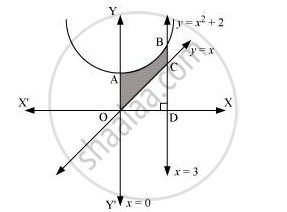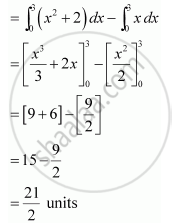# Find the Area of the Region Bounded by the Curves Y = X2 + 2, Y = X, X = 0 and X = 3 - Mathematics

Find the area of the region bounded by the curves y = x+ 2, xx = 0 and x = 3

#### Solution

The area bounded by the curves, y = x+ 2, xx = 0, and x = 3, is represented by the shaded area OCBAO asThen, Area OCBAO = Area ODBAO – Area ODCOIs there an error in this question or solution?
Chapter 8: Application of Integrals - Exercise 8.2 [Page 371]

#### APPEARS IN

NCERT Class 12 Maths
Chapter 8 Application of Integrals
Exercise 8.2 | Q 3 | Page 371

Share